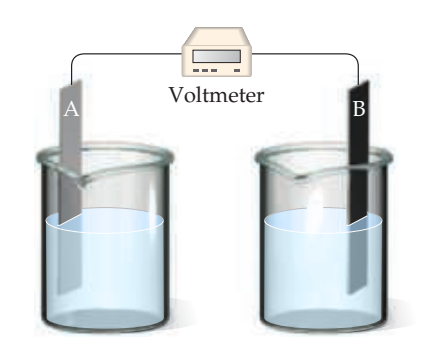×
Get Full Access to Chemistry: The Central Science - 14 Edition - Chapter 20 - Problem 20.4
Get Full Access to Chemistry: The Central Science - 14 Edition - Chapter 20 - Problem 20.4

×ISBN: 9780134414232 1274

## Solution for problem 20.4 Chapter 20

Chemistry: The Central Science | 14th Edition

• Textbook Solutions
• 2901 Step-by-step solutions solved by professors and subject experts
• Get 24/7 help from StudySoup virtual teaching assistantsChemistry: The Central Science | 14th Edition

4 5 1 321 Reviews
25
5
Problem 20.4

Assume that you want to construct a voltaic cell that uses the following half-reactions:

$$\mathrm{A}^{2+}(a q)+2 \mathrm{e}^{-} \longrightarrow \mathrm{A}(s) \quad E_{\text {red }}^{\circ}=-0.10 \mathrm{~V}$$

$$\mathrm{B}^{2+}(a q)+2 \mathrm{e}^{-} \longrightarrow \mathrm{B}(s) \quad E_{\mathrm{red}}^{\circ}=-1.10 \mathrm{~V}$$

You begin with the incomplete cell pictured here in which the electrodes are immersed in water.(a) What additions must you make to the cell for it to generate a standard emf?

(b) Which electrode functions as the cathode?

(c) Which direction do electrons move through the external circuit?

(d) What voltage will the cell generate under standard conditions? [Sections 20.3 and 20.4]

Text Transcription:

{A}^{2+}(a q)+2 {e}^{-} \longrightarrow {A}(s) \quad E_red }}^{\circ}=-0.10 V

{B}^{2+}(a q)+2 {e}^{-} \longrightarrow {B}(s) \quad E_{red}}^{\circ}=-1.10 V

Step-by-Step Solution:
Step 1 of 3

1 Chapter 3 The Mole and Stoichiomtery Objectives: Avogadro's number Moles Percent Composition of Compounds Empirical Formula Stoichiometry Limiting Reagent Reaction Yield ­A dime weighs 2.27 g. The mass of a bag of dimes is 227 g. How many dimes are in the bag How do you determine the chemical formula of a substance How much of Y can you prepare from a given quantity of X X (aq)  Y (aq) Molecular Weight and Formula Weight: ­Molecular weight is the sum of the atomic weights of all atoms in a molecule. Ex: What is the molecular weight of H O 2 2 34.0147 g/mol The formula weight (FW) of a substance is the sum of the atomic weights of all atoms in a formula unit of the compound whether molecular or not. Ex: What is the formula weight of H S2 4 98.079 g/mol Moles: 2 ­Mole (mol)­ Latin, a large number A very important unit in chemistry ­A 10.0 g sample of ethanol (<3 teaspoons) contains: 131,000,000,000,000,000,000,000 molecules. It is not very easy to count all these molecules! ­One mole is defined as the number of atoms in exactly 12 g of C­12 atoms. 1 mole Na = 23.00 g Na ­Mole concept to deal w/ the number of molecules. mole = mass molecular weight ­One mole of NH c3ntains 17.04g of the molecule. Avogadro's Number: ­A special unit to describe a very l

Step 2 of 3

Step 3 of 3

## Discover and learn what students are asking

Chemistry: The Central Science : Transition Metals and Coordination Chemistry
?True or false? The following ligand can act as a bidentate ligand?

Chemistry: The Central Science : The Chemistry of Life: Organic and Biological Chemistry
?Indicate whether each statement is true or false. (a) Butane contains carbons that are $$s p^{2}$$ hybridized. (b) Cyclohexane is

Unlock Textbook Solution